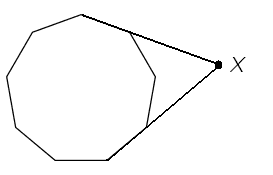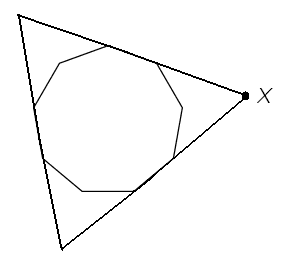#### You may also like### Consecutive Numbers

An investigation involving adding and subtracting sets of consecutive numbers. Lots to find out, lots to explore.### Calendar Capers

Choose any three by three square of dates on a calendar page...### Days and Dates

Investigate how you can work out what day of the week your birthday will be on next year, and the year after...

# Outside the Nonagon

##### Age 11 to 14 ShortChallenge LevelThe exterior angles of a regular nonagon are $360^{\circ}\div 9 = 40^{\circ}$, whence the interior angles are $180^{\circ} - 40^{\circ}= 140 ^{\circ}$.

In the arrowhead quadrilateral whose rightmost vertex is X, three of the angles are $40^{\circ}$, $40^{\circ}$ and $360^{\circ} - 140^{\circ}=220^{\circ}$ and these add up to $300^{\circ}$.

So the angle at X is $60^{\circ}$.

[It is now posible to see that the entire nonagon can fit neatly inside an equilateral triangle and so the angle X is $60^{\circ}$ ]This problem is taken from the UKMT Mathematical Challenges.
You can find more short problems, arranged by curriculum topic, in our short problems collection.